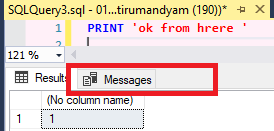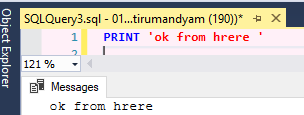# ALTER VIEW must be the only statement in the batch

I'm getting the above error but can't see why?
If I uncomment the GO above to start a new batch I get a syntax error.
What am I doing wrong?
Code below:
USE ProjectsEngineering;
GO
IF object_id('dbo.sp_BayNodes') IS NOT NULL
DROP PROC dbo.sp_BayNodes
GO

CREATE PROCEDURE dbo.sp_BayNodes @projID VARCHAR(10),@EVersion VARCHAR(10)
AS
BEGIN
--SET NOCOUNT ON;
--GO

ALTER VIEW dbo.v_Nodes
AS
SELECT DISTINCT
s.SiteName + e.VoltValue + '_' + c.CircuitName AS BayName
FROM ProjectEntries e
LEFT JOIN ProjectCircuits c
ON e.CircuitID = c.ID
LEFT OUTER JOIN ProjectSubstations s
ON e.SubstationID = s.ID
WHERE e.projectID = @projID
AND e.EndVersion = @EVersion
AND e.SCS_EventStatus != 'SPARE'
AND((NGCC = + 'Y' + ) OR(IndNGCC = 'Y' + ))
AND(CIndIEC1 != '-' OR CIndIEC2 != '-' OR IEC101 != '-')
AND LEFT(c.CircuitName, 1) != 'X' --Ignore Plant
AND c.CircuitName != 'ARS' AND c.CircuitName != 'ATCC';
END
GO

Look at the above and see what is wrong. Why do you have an unfinished stored procedure and then an Alter view

because I want the stored proc to create the view or is this not possible like this?
I have tried wrapping the Alter View in dynamic SQL:USE ProjectsEngineering;
GO
IF object_id('dbo.sp_BayNodes') IS NOT NULL
DROP PROC dbo.sp_BayNodes
GO

CREATE PROCEDURE dbo.sp_BayNodes @projID AS VARCHAR(10), @EVersion AS VARCHAR(10)
AS
BEGIN
--SET NOCOUNT ON;
DECLARE @SQL NVARCHAR(MAX)

SET @SQL = '
ALTER VIEW dbo.v_Nodes
AS
SELECT DISTINCT
CASE
WHEN(IEC101 != ''-'') AND CHARINDEX(''-'', IEC101) = 3 THEN CAST(LEFT(IEC101, 2) AS INT)
WHEN(IEC101 != ''-'') AND CHARINDEX(''-'', IEC101) = 2 THEN CAST(LEFT(IEC101, 1) AS INT)
WHEN(CIndIEC1 != ''-'') AND CHARINDEX(''-'', CIndIEC1) = 3 THEN CAST(LEFT(CIndIEC1, 2) AS INT)
WHEN(CIndIEC1 != ''-'') AND CHARINDEX(''-'', CIndIEC1) = 2 THEN CAST(LEFT(CIndIEC1, 1) AS INT)
WHEN(CIndIEC2 != ''-'') AND CHARINDEX(''-'', CIndIEC2) = 3 THEN CAST(LEFT(CIndIEC2, 2) AS INT)
WHEN(CIndIEC2 != ''-'') AND CHARINDEX(''-'', CIndIEC2) = 2 THEN CAST(LEFT(CIndIEC2, 1) AS INT)
END AS IEC_Node_No,
s.SiteName + e.VoltValue + ''_'' + c.CircuitName AS BayName
FROM ProjectEntries e
LEFT JOIN ProjectCircuits c
ON e.CircuitID = c.ID
LEFT OUTER JOIN ProjectSubstations s
ON e.SubstationID = s.ID
WHERE e.projectID = @projID
AND e.EndVersion = @EVersion
AND e.SCS_EventStatus != ''SPARE''
AND((NGCC = + ''Y'' + ) OR(IndNGCC = ''Y'' + ))
AND(CIndIEC1 != ''-'' OR CIndIEC2 != ''-'' OR IEC101 != ''-'')
AND LEFT(c.CircuitName, 1) != ''X'' --Ignore Plant
AND c.CircuitName != ''ARS'' AND c.CircuitName != ''ATCC''';

``````		EXEC sp_executesql @SQL;
``````

END
GO

This complies ok but when I run it using:
USE ProjectsEngineering
GO
EXEC sp_BayNodes @projID = '568', @EVersion = '28.4'

I get an error:Msg 137, Level 15, State 2, Procedure v_Nodes, Line 19 [Batch Start Line 2]
Must declare the scalar variable "@projID".
So I must be doing something wrong with this too. Please excuse my lack of SQL knowledge as I'm fairly new, but any help with this appreciated.

hi

i think the problem is here !!! you have to do it like this !!

SET
@SQL
= ' alter '
+@projID+
' select ok from table '+
+@EVersion+
'rest of SQL Statement'

OK, I've altered my code to what you suggest(I think):
USE ProjectsEngineering;
GO
IF object_id('dbo.sp_BayNodes') IS NOT NULL
DROP PROC dbo.sp_BayNodes
GO

CREATE PROCEDURE dbo.sp_BayNodes @projID AS VARCHAR(10), @EVersion AS VARCHAR(10)
AS
BEGIN
--SET NOCOUNT ON;
DECLARE @SQL NVARCHAR(MAX)

SET @SQL = '
ALTER VIEW dbo.v_Nodes
AS
SELECT DISTINCT
CASE
WHEN(IEC101 != ''-'') AND CHARINDEX(''-'', IEC101) = 3 THEN CAST(LEFT(IEC101, 2) AS INT)
WHEN(IEC101 != ''-'') AND CHARINDEX(''-'', IEC101) = 2 THEN CAST(LEFT(IEC101, 1) AS INT)
WHEN(CIndIEC1 != ''-'') AND CHARINDEX(''-'', CIndIEC1) = 3 THEN CAST(LEFT(CIndIEC1, 2) AS INT)
WHEN(CIndIEC1 != ''-'') AND CHARINDEX(''-'', CIndIEC1) = 2 THEN CAST(LEFT(CIndIEC1, 1) AS INT)
WHEN(CIndIEC2 != ''-'') AND CHARINDEX(''-'', CIndIEC2) = 3 THEN CAST(LEFT(CIndIEC2, 2) AS INT)
WHEN(CIndIEC2 != ''-'') AND CHARINDEX(''-'', CIndIEC2) = 2 THEN CAST(LEFT(CIndIEC2, 1) AS INT)
END AS IEC_Node_No,
s.SiteName + e.VoltValue + ''_'' + c.CircuitName AS BayName
FROM ProjectEntries e
LEFT JOIN ProjectCircuits c
ON e.CircuitID = c.ID
LEFT JOIN ProjectSubstations s
ON e.SubstationID = s.ID
WHERE e.projectID = ' + @projID +
' AND e.EndVersion = ' + @EVersion +
' AND e.SCS_EventStatus != ''SPARE''
AND ((NGCC = + ''Y'' + ) OR (IndNGCC = ''Y'' + ))
AND ((CIndIEC1 != ''-'') OR (CIndIEC2 != ''-'' ) OR (IEC101 != ''-''))
AND LEFT(c.CircuitName, 1) != ''X''
AND c.CircuitName != ''ARS''
AND c.CircuitName != ''ATCC''';

``````		EXEC sp_executesql @SQL;
``````

END
GO
This compiles without error.
When I run:
USE ProjectsEngineering
GO
EXEC dbo.sp_BayNodes @projID = '568', @EVersion = '28.4'
GO
I get error:Msg 102, Level 15, State 1, Procedure v_Nodes, Line 20 [Batch Start Line 2]
Incorrect syntax near ')'.
I cannot see anything wrong with my syntax and the select statement runs ok in isolation.

first try

print @SQL ....... instead of execute sp_execute @SQL

see whats coming in print .....the statement
that will show whats the problem ...

Changed as per your suggestion and when I run the sp I get:

ALTER VIEW dbo.v_Nodes
AS
SELECT DISTINCT
CASE
WHEN(IEC101 != '-') AND CHARINDEX('-', IEC101) = 3 THEN CAST(LEFT(IEC101, 2) AS INT)
WHEN(IEC101 != '-') AND CHARINDEX('-', IEC101) = 2 THEN CAST(LEFT(IEC101, 1) AS INT)
WHEN(CIndIEC1 != '-') AND CHARINDEX('-', CIndIEC1) = 3 THEN CAST(LEFT(CIndIEC1, 2) AS INT)
WHEN(CIndIEC1 != '-') AND CHARINDEX('-', CIndIEC1) = 2 THEN CAST(LEFT(CIndIEC1, 1) AS INT)
WHEN(CIndIEC2 != '-') AND CHARINDEX('-', CIndIEC2) = 3 THEN CAST(LEFT(CIndIEC2, 2) AS INT)
WHEN(CIndIEC2 != '-') AND CHARINDEX('-', CIndIEC2) = 2 THEN CAST(LEFT(CIndIEC2, 1) AS INT)
END AS IEC_Node_No,
s.SiteName + e.VoltValue + '_' + c.CircuitName AS BayName
FROM ProjectEntries e
LEFT JOIN ProjectCircuits c
ON e.CircuitID = c.ID
LEFT JOIN ProjectSubstations s
ON e.SubstationID = s.ID
WHERE e.projectID = 568 AND e.EndVersion = 28.4 AND e.SCS_EventStatus != 'SPARE'
AND ((NGCC = + 'Y' + ) OR (IndNGCC = 'Y' + ))
AND ((CIndIEC1 != '-') OR (CIndIEC2 != '-' ) OR (IEC101 != '-'))
AND LEFT(c.CircuitName, 1) != 'X'
AND c.CircuitName != 'ARS'
AND c.CircuitName != 'ATCC'

Completion time: 2020-05-12T16:15:53.4278932+01:00
As you can see, no errors highlighted. If I change back to EXEC sp_executesql @SQL; the same error occurs?

print @SQL prints out the select statement

in

TSQL ..Messages tab .. you have to examine the SQL statement from thereThe SQL that I sent in my previous post was from the Messages tab.

hi

another thing is in set @SQL = ' select 'name' '

inside the main quotes ' '
all these single quotes ' have to be double quotes ''

set @SQL = ' select 'name' '
this becomes
set @SQL = ' select ''name'' '

Why do you want a stored procedure to create a view that only changes values of a parameter?

Really???
AND ((NGCC = + 'Y' + ) OR (IndNGCC = 'Y' + ))

Try:

``````SET ANSI_NULLS, QUOTED_IDENTIFIER, ANSI_PADDING ON;
GO
CREATE PROCEDURE dbo.sp_BayNodes
@projID AS varchar(10)
,@EVersion AS varchar(10)
AS
SET NOCOUNT, XACT_ABORT ON;
DECLARE @SQL NVARCHAR(MAX);

SET @SQL = 'ALTER VIEW dbo.v_Nodes
AS
-- All columns should have an alias!!!
SELECT DISTINCT
CASE
WHEN IEC101 != ''-'' AND CHARINDEX(''-'', IEC101) = 3
THEN CAST(LEFT(IEC101, 2) AS int)
WHEN IEC101 != ''-'' AND CHARINDEX(''-'', IEC101) = 2
THEN CAST(LEFT(IEC101, 1) AS int)
WHEN CIndIEC1 != ''-'' AND CHARINDEX(''-'', CIndIEC1) = 3
THEN CAST(LEFT(CIndIEC1, 2) AS int)
WHEN CIndIEC1 != ''-'' AND CHARINDEX(''-'', CIndIEC1) = 2
THEN CAST(LEFT(CIndIEC1, 1) AS int)
WHEN CIndIEC2 != ''-'' AND CHARINDEX(''-'', CIndIEC2) = 3
THEN CAST(LEFT(CIndIEC2, 2) AS int)
WHEN CIndIEC2 != ''-'' AND CHARINDEX(''-'', CIndIEC2) = 2
THEN CAST(LEFT(CIndIEC2, 1) AS int)
END AS IEC_Node_No,
s.SiteName + e.VoltValue + ''_'' + c.CircuitName AS BayName
FROM ProjectEntries e
LEFT JOIN ProjectCircuits c
ON e.CircuitID = c.ID
LEFT JOIN ProjectSubstations s
ON e.SubstationID = s.ID
WHERE e.projectID = @projID
AND e.EndVersion = @EVersion
AND e.SCS_EventStatus != ''SPARE''
AND (NGCC = ''Y'' OR IndNGCC = ''Y'')
AND (CIndIEC1 != ''-'' OR CIndIEC2 != ''-'' OR IEC101 != ''-'')
AND LEFT(c.CircuitName, 1) != ''X''
AND c.CircuitName != ''ARS''
AND c.CircuitName != ''ATCC'';';

--print @SQL
EXEC sp_executesql @SQL, '@projID AS varchar(10), @EVersion AS varchar(10)', @projID, @EVersion;
GO ``````

I cut and pasted your code into SSMS and it compiled without error. However, when I run the sp I get:
Msg 214, Level 16, State 3, Procedure sp_executesql, Line 1 [Batch Start Line 2]
Procedure expects parameter '@params' of type 'ntext/nchar/nvarchar'.

Completion time: 2020-05-12T18:28:50.8879230+01:00

have you tried the double quotes i was telling you

This could be the cause of errors

Double quotes could be the reason for your errors

Cracked it!
What I did was temporarily comment out most of the where clause except for the parameters, which worked. I then uncommented each line one at a time until the whole prog worked. For each line, it was a matter of when to use a combination of quotes, how many, position, +sign.
Thank you very much for your help in this matter. It's only taken me 3 days to get this working!
Working code below:
GO
USE ProjectsEngineering;
GO
IF object_id('dbo.sp_BayNodes') IS NOT NULL
DROP PROC dbo.sp_BayNodes
GO

CREATE PROCEDURE dbo.sp_BayNodes @projID AS VARCHAR(10), @EVersion AS VARCHAR(10)
AS
BEGIN
SET NOCOUNT, XACT_ABORT ON;
DECLARE @SQL NVARCHAR(MAX)

SET @SQL = '
ALTER VIEW dbo.v_Nodes
AS
SELECT DISTINCT
CASE
WHEN(IEC101 != ''-'') AND CHARINDEX(''-'', IEC101) = 3 THEN CAST(LEFT(IEC101, 2) AS INT)
WHEN(IEC101 != ''-'') AND CHARINDEX(''-'', IEC101) = 2 THEN CAST(LEFT(IEC101, 1) AS INT)
WHEN(CIndIEC1 != ''-'') AND CHARINDEX(''-'', CIndIEC1) = 3 THEN CAST(LEFT(CIndIEC1, 2) AS INT)
WHEN(CIndIEC1 != ''-'') AND CHARINDEX(''-'', CIndIEC1) = 2 THEN CAST(LEFT(CIndIEC1, 1) AS INT)
WHEN(CIndIEC2 != ''-'') AND CHARINDEX(''-'', CIndIEC2) = 3 THEN CAST(LEFT(CIndIEC2, 2) AS INT)
WHEN(CIndIEC2 != ''-'') AND CHARINDEX(''-'', CIndIEC2) = 2 THEN CAST(LEFT(CIndIEC2, 1) AS INT)
END AS IEC_Node_No,
s.SiteName + e.VoltValue + ''_'' + c.CircuitName AS BayName
FROM ProjectEntries e
LEFT JOIN ProjectCircuits c
ON e.CircuitID = c.ID
LEFT JOIN ProjectSubstations s
ON e.SubstationID = s.ID
WHERE e.projectID = ' + @projID +
' AND e.EndVersion = ' + @EVersion +
' AND e.SCS_EventStatus != ''SPARE''
AND (NGCC = ''Y'' OR IndNGCC = ''Y'')
AND ((CIndIEC1 != ''-'') OR (CIndIEC2 != ''-'' ) OR (IEC101 != ''-''))
AND LEFT(c.CircuitName, 1) != ''X''
AND c.CircuitName != ''ARS''
AND c.CircuitName != ''ATCC''' + ';'

``````		EXEC sp_executesql @SQL;
``````

END
GO

Could you explain why you are doing this?

I'm doing this to query the database and produce a view which can be accessed by c#. Parameters passed by c# will determine the data that is in the view.

So why not make it much simpler and just have C# execute a stored procedure and return the data based on the parameters? No need for dynamically changing a view each time the parameters are changed.

In fact - doing this is going to cause concurrency issues in your application. Only one person can execute that part of the code at a time and will be blocked if someone else is currently executing the code.

2 Likes

you are going to run into some major issues you will later regret. why not call the sproc from c#

``````exec sp_BayNodes @parm1 = 'Chicken'

create proc sp_BayNodes
as
begin
select x,yz,
from dbo.v_Nodes
where foo = @parm1

end
``````

and you will also avoid sql injection, if someone purposefully sends,
`DELETE database main `

1 Like